Next: Implementation Details Up: Optimal Regular Volume Sampling Previous: Introduction

Subsections

# Baseband Optimal Sampling

Figure 2: 2D regular rectangular and hexagonal sampling in the spatial and frequency domains.
 spatial domain frequency domain rectangular sampling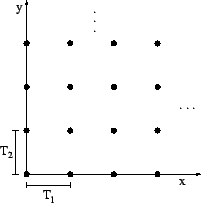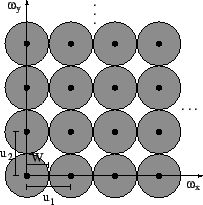hexagonal sampling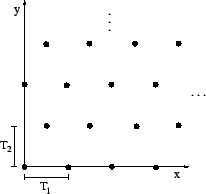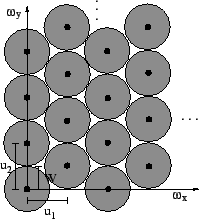Usually, no a priori knowledge is available about the (continuous) underlying functions we are sampling. Therefore, we assume that these functions are isotropic, i.e., that they do not have a preferred direction. Another common assumption is that they are band-limited. Both assumptions together result in the property that the frequency responses of such functions are hyper-spheres (circles in 2D and spheres in 3D, respectively).

Sampling such spherically band-limited functions results in replicating the primary spectrum in the frequency domain . In order to reconstruct the underlying continuous signal perfectly, we need to ensure that the samples in spatial domain are close enough, so that the aliased spectra in the frequency domain do not overlap. Optimal sampling is achieved when the number of samples that fulfill this condition is minimal. In 1D this is also known as the Nyquist sampling rate. In order to optimally sample in higher dimensions (i.e., to use as few samples as possible), aliased spectra in the frequency domain have to be packed as closely as possible. This problem is known as the sphere packing problem , which has been extensively studied and solutions for regular packing structures in 2D and 3D exist.

As a motivation and for the sake of simplicity we will first describe our method to find an optimal sampling pattern in 2D before we delve into the mysterious structure of 3D Euclidian space.

## Optimal sampling density in 2D

We will describe sampling as a mapping from indices to actual sample positions :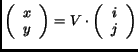(1)

Here the integers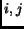are the indices of the sample and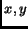is the corresponding sampling position. The matrix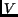, which is called sampling matrix, describes the mapping itself, e.g.,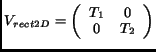(2)

is the matrix for rectangular sampling which simplifies to the commonly used regular (Cartesian) sampling for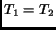. Hexagonal sampling is most conveniently described by the matrix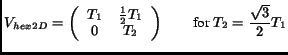(3)

which virtually just performs a shear of the rectangular samples followed by a for-shortening. Whendescribes the sampling in spatial domain, the matrix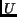, satisfying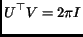(4)

with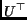being the transpose ofand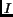being the identity matrix, describes the positions of the replicas in frequency domain.is therefore called the periodicity matrix . Applying Eq. 4 to Eq. 2 we obtain the periodicity matrix for rectangular sampling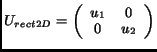(5)

with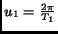and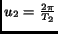. The periodicity matrix for hexagonal sampling is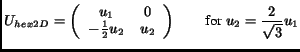(6)

where again. The 2D Fourier Transform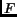of a circularly band-limited signal has the property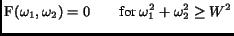(7)

where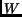is the maximum frequency in the data set. This baseband can be inscribed, for example, in a square with length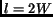(corresponding to rectangular sampling). In other words,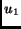and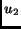in Eq. 5 have to be equal to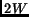. On the other hand, the baseband can also be inscribed in a hexagon with side length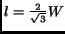(corresponding to hexagonal sampling). This means that in Eq. 6must be equal to(see Fig. 2). Calculating the sampling matrices from these periodicity matrices, we end up with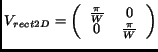(8)

where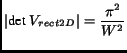(9)

and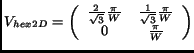(10)

where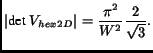(11)

The sampling density is proportional to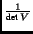. By taking the ratio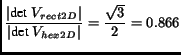(12)

we see that hexagonal sampling requires 13.4% fewer samples than rectangular sampling.

## Optimal sampling density in 3D

Analogous to the 2D case, we can describe the mapping from indices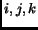to coordinates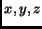for rectilinear grids using the following matrix: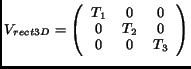(13)

which is regular (rectangular) when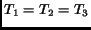.

As expected, regular rectangular sampling in 3D is (as in 2D) not optimal. It is important to note that an optimal sphere packing for arbitrary packing structures in 3D is not known. However, several optimal packing structures, all with equal sampling density, are known for the case of regular sampling, i.e., structures that can be described by three base vectors. Fortunately, this is exactly what we need, since we do not want to sacrifice the implicit indexing of the grid points that makes regular grid representations so attractive.

Among the optimal regular packing structures are the hexagonal close packed (hcp) structure and the face centered cubic (fcc) structure . In order to achieve a close packing in the frequency domain using an fcc lattice (the reason why not using an hcp lattice is explained at the end of this section), we use the following matrix: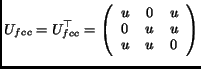(14)

An fcc lattice consists of simple cubic cells with additional sampling points in the center of each cell face. One cell of an fcc lattice is depicted in Fig. 3 with the base vectors from Eq. 14.

By plugging Eq. 14 in Eq. 4 we end up with a sampling matrix in spatial domain which describes a body centered cubic (bcc) lattice: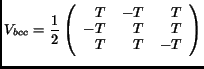(15)

with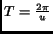.

A bcc lattice also consists of a simple cubic cell but with only one additional sampling point, which is right in the center of the cell. Fig. 4 depicts one cell of a bcc lattice. Note, that the base vectors of Fig. 4 do not correspond to Eq. 15, as these are rather unintuitive. We chose another set of base vectors, which is more convenient for our purpose of indexing the data points (see Section 3.1). They are described by the sampling matrix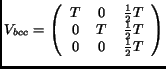(16)

It is easily verified that the sampling matrices in Eqs. 15 and 16 describe the same set of sample points.

To guarantee that the replicas in frequency domain do not overlap,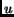must be equal tofor rectangular sampling. Since the periodicity matrix is analogous to the 2D periodicity matrix we end up with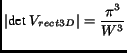(17)

For the fcc lattice,must be equal to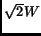, which yields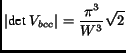(18)

By again taking the ratio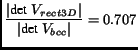(19)

we see that we need 29.3% fewer samples than with rectangular sampling. This means that if we sample a function on a regular rectangular grid with sampling distance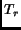, we can increase the sampling distance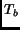for a bcc grid to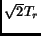.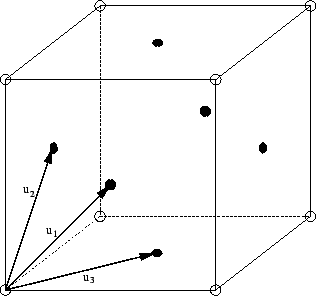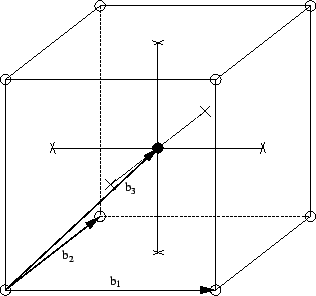In the above example we started with an fcc lattice in the frequency domain which resulted in a bcc lattice in the spatial domain. We could also choose an hcp lattice, since it has the same packing density as an fcc lattice . However, an hcp lattice in the frequency domain is also an hcp lattice in the spatial domain and hcp lattices are rather difficult to index . Therefore, we prefer to use a bcc grid.Next: Implementation Details Up: Optimal Regular Volume Sampling Previous: Introduction
Thomas Theußl 2001-08-05